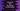# 5 different JavaScript programs to find the average of array values## How to find the average of the array values in JavaScript:

In this post, we will learn how to find the average of all the items of an array in JavaScript. To find the average of n numbers, we need to find the sum of the numbers and divide it by n. So, total sum/n is the average of n numbers.

To find the average of array numbers, we have to iterate over the array items one by one. JavaScript provides different ways to iterate over an array. We can also use the reduce method of JavaScript array to find the required average.

### Method 1: By using a for loop:

This is the traditional approach to iterate an array. The for loop will use an index to iterate over the array elements. The program will use this loop to find the sum of all the numbers of the array. It will divide the sum by the total numbers to calculate the average.

``````let arr = [1, 2, 3, 4, 5, 6, 7];
let sum = 0;

for (let i = 0; i < arr.length; i++) {
sum += arr[i];
}

const average = sum / arr.length;

console.log(`Average: \${average}`);``````

Here,

• arr is the given array.
• The for loop runs from i = 0 to i = array length - 1.
• On each step, it adds the array element at index i to the sum variable. This variable is initialized as 0.
• The average is calculated by dividing the sum by the length of the array.
• The last line is printing the average value.

### Method 2: By using while loop:

The above program can be changed to use a while loop instead of a for loop. It will work in a similar way. The loop will check the condition and execute its body if the condition is true.

``````let arr = [1, 2, 3, 4, 5, 6, 7];
let sum = 0;
let i = 0;

while (i < arr.length) {
sum += arr[i];
i++;
}

const average = sum / arr.length;

console.log(`Average: \${average}`);``````

It will print the same result.

### Method 3: By using for..of loop:

We can use also use a for..of loop to iterate over the array items. The for..of loop can iterate over the array elements directly. It doesn’t need the index.

``````let arr = [1, 2, 3, 4, 5, 6, 7];
let sum = 0;

for (let item of arr) {
sum += item;
}

const average = sum / arr.length;

console.log(`Average: \${average}`);``````

We don’t have to create another variable to hold the index. It will print the same result.

### Method 4: By using forEach:

forEach function is another way to iterate over an array items. The advantage of this function is that we can pass an arrow function as its parameter, which makes the code concise and clean.

``````let arr = [1, 2, 3, 4, 5, 6, 7];
let sum = 0;

arr.forEach(item => sum+=item);

const average = sum / arr.length;

console.log(`Average: \${average}`);``````

You can also pass a normal function to forEach.

### Method 5: By using reduce:

The reduce() function takes one function as its argument and executes that function on each element of the array. It is called reducer function.

The reduce is an inbuilt array function. It passes the calculated value to the next execution. It also takes one initial value that is passed to the first execution.

We can use the reduce function to find the sum of all numbers of an array.

``````let arr = [1, 2, 3, 4, 5, 6, 7];
let sum = arr.reduce((accumulator, current) => accumulator + current, 0);

const average = sum / arr.length;

console.log(`Average: \${average}`);``````

Here,

• The initial item is 0.
• The reducer function takes the accumulator, which is the result of the last execution and the current number as its parameters and returns the sum of both. On first execution, the value of accumulator is 0.
• Once it iterates over all of the numbers, it returns the sum of the array values.
• Similar to the above examples, we can divide this sum by the length of the array to find the average.

If you run this program, it will print the same result.

``Average: 4``